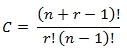# SQL Server COMBINA function

COMBINA
Updated: 25 Apr 2017

Use the scalar function COMBINA to calculate the number of combinations with repetition of n things taken r at a time. The formula is:Syntax
SELECT [wct].[COMBINA] (
<@Number, int,>
,<@Number_chosen, int,>)
Arguments
 Input Name Description @Number Number of things. @Number must be of type int or of a type that implicitly converts to int. @Number_chosen Number to be taken. @Number_chosen must be of type int or of a type that implicitly converts to int.
Return Type
float
Remarks
Examples
Example #1
SELECT
wct.COMBINA(4,2) as COMBINA

This produces the following result.Copyright 2008-2023 Westclintech LLC         Privacy Policy        Terms of Service